## Friday, March 8, 2013

### Why is Wait-Free so important?

#### Lock-Free (full synchronization)

Imagine a Lock-Free algorithm or data-structure with a single function, where this function spends all of its time doing synchronization.
Let's say that each function call in single threaded mode takes 10 ns, and with every added thread that calls the same function, we will get contention on the synchronized state (variables).
It is easy to see, that if you have a single thread, the average number of times the function is called and returns, is 1 every 10 nanoseconds. Let's call this: the number of tasks per 10 ns. If you add more threads, they will increase the time the other threads take to complete, because only one will be able to win the consensus.
Remember that Lock-Free gives guarantee that one thread is making a progress, but it doesn't say which one, and in this particular case, every time one thread makes a progress (completes a task) the other threads will have to start over: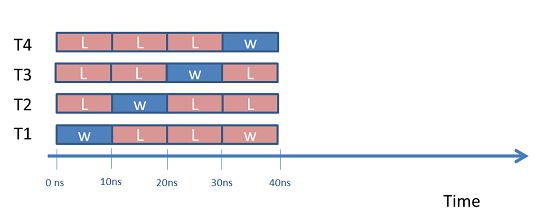In the plot, W means that the thread has won and L stands for a loss, that implies a retry.

#### Lock-Free (small synchronization)

The above schematic doesn't accurately describe most of the Lock-Free algorithms in existence because, they don't spend all of their time doing synchronization, instead, the largest slice of time is usually spent doing some kind of calculation related to the algorithm itself, which doesn't necessarily require re-computation in case of another thread winning, and only a portion of the time is actually spent doing the synchronization.
As such, let us imagine a case where the function takes 10 ns to complete its task, but only the first 2.5 nanoseconds are spent doing synchronization. It is easy to see that on average, per 10 ns, we could have multiple tasks running concurrently on multiple threads/cores, and up to 4 threads would be able to run simultaneously, as shown in the schematic below: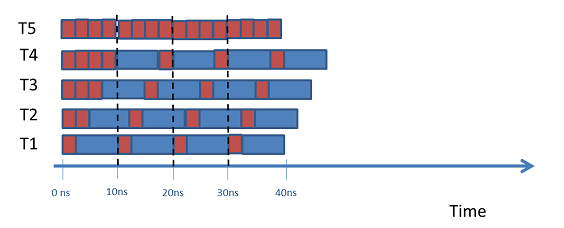In the example described above, the synchronization time takes (at least) a quarter of the 10 ns of the time spent by the function.
Moreover, if draw a plot of the overall performance of this algorithm as the number of threads increases, we would see something that scales almost linearly up to 4 threads, and then hits a ceiling and doesn't go above it, and may even reduce the performance if the number of threads is further increased: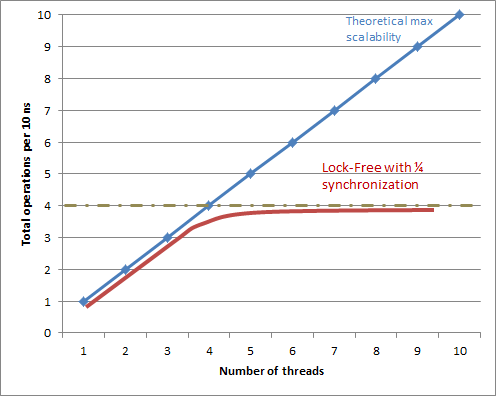Notice we're assuming that we have more than 4 cores where we can run these 4 or more threads.

#### Wait-Free-Population-Oblivious

Consider now a different scenario, where the algorithm we have is Wait-Free-Population-Oblivious, or WFPO for short. Let us say that the algorithm is 3 times slower that the previous Lock-Free algorithm, such that it takes 30ns to complete a single task, but it is WFPO which by definition means that, as long as you have enough cores, all threads are guaranteed to make progress, and because it is WFPO and not just Wait-Free, the number of extra instructions does not depend on the number of threads and, therefore, remains (more or less) constant as the number of threads increases.
The schematic would like like this: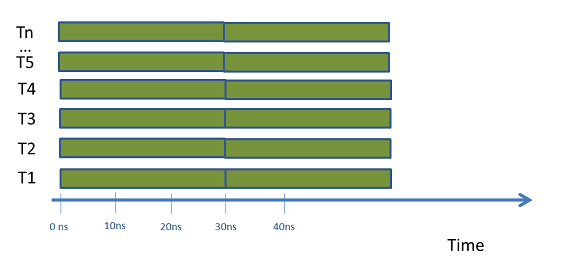The plot of the overall performance of this algorithm as the number of threads increases can be seen below: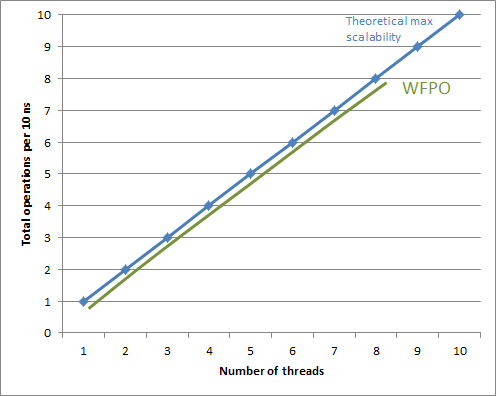Notice that the performance keeps on scaling until you run our of cores to run the new threads, or the synchronization primitives you are using, stop being WFPO due to some hardware limitation.

#### Algorithm optimization

Let's go back to the Lock-Free scenario with a synchronization during 1ns and imagine that some smart guy was able to optimize the time it takes to do the calculation, and now instead of taking 7.5 ns, it takes just 2.5 ns, which means that in single-thread mode the performance of the algorithm has just doubled. How much performance would we gain for the multi-threaded code?
The answer is not trivial, and it is 0. The reason why it is zero, is because we can only run threads while there is no contention, so even if the rest of the code runs faster, each thread must still have to contend with the other threads and only one will win, while the other will have to contend again: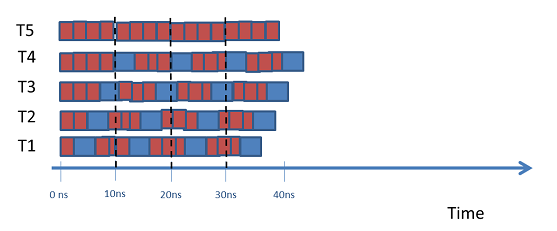For the WFPO algorithm, if you manage to cut 5ns out of the computation portion, you get a performance increase of about 17% overall (5 ns / 30 ns) .

Now you say: Well, what if we can optimize the synchronization code in the Lock-Free algorithm so that goes from taking 2.5 ns to spending only 1 ns?
Yeah, you could do that, but you would probably have to re-design the whole synchronization part, which is the same as saying you made up a new Lock-Free consensus mechanism, which is in itself a publishable result and not an easy feat (to say the least). Only guys like Maurice Herlihy, Nir Shavit, or Doug Lea, are capable of that kind of thing... are you up to the task?   ;)

#### Wait-Free

How about vanilla Wait-Free (non Population-Oblivious)?
Well, it depends: if the number of operations grows very gently with the number of threads, then the performance may scale and increase.
If the number of operations increases quadratically with the number of threads, then you're probably out of luck, and that algorithm won't be much better than a Lock-Free one (or may be even worse).
Basically, non-Population-Oblivious Wait-Free algorithms and data-structures will have a behavior somewhere between the Lock-Free ones and the WFPOs.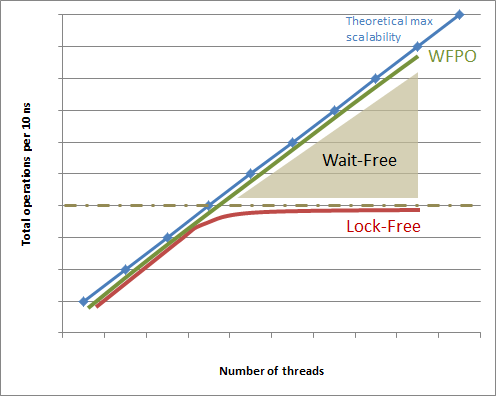#### Conclusion

Lock-Free algorithms and data-structures have an intrinsic scalability ceiling, which means that at a certain point, no matter how many more threads/cores you throw at the problem, it will not improve the overall performance and may in fact decrease it due to high contention
Adding more threads to a Lock-Free algorithm is good only to a certain point, and you would be surprised of how low that limit can be for certain algorithms and data-structures. If you're thinking of the future, or your application is meant to run on a large number of threads/cores, then aim for Wait-Free-Population-Oblivious, otherwise you're not going very far.

Summarizing in one sentence, forget about Lock-Free and regular Wait-Free, if you want to design an algorithm that scales for a large number of threads, you need something that is Wait-Free-Population-Oblivious!

Actually, the title of this post should have been: "Why is Wait-Free-Population-Oblivious so important"?